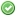rfc:pow-operator

This is an old revision of the document!

PHP RFC: Power Operator

Introduction

This proposal is three-fold:

1. Turn the existing pow() function into a language construct.
2. Introduce an exponential (right associative) operator **.
• Easier to read and shorter to write.
• Can be found in other languages.
3. Introduce an exponential assignment operator **=

Proposal

pow() as language construct

No changes to existing code, safe for one:

var_dump(pow([], 2)); // int(0) instead of float(0)

Power operator

A short working example:

echo 2 ** 3; // 8

\$base = gmp_init(2);
\$exponent = 3;
var_dump(\$base ** \$exponent);

// output
object(GMP)#3 (1) {
["num"]=>
string(1) "8"
}

Example of exponent assignment:

\$x = 2;
\$x **= 3;
echo \$x; // 8

Important

The proposed associativity is right, just like how power towers work.

The operator precedence is:

• higher than the bitwise not (~),
• lower than array dereferencing,
• lower than unary minus.

Examples:

echo 2 ** 3 ** 2; // 512 (not 64)
echo -3 ** 2; // 9 (not -9)
echo ~3 ** 2; // -10 (not 16)

Discussion

Should 2 ** 3 ** 2 yield 64 (left associative), 512 (right associative) or throw an error (non associative)?

The exponent operator evaluation order should be based on Tetration and therefore be right associative.

Languages with left associative exponential operator

• VB (not by choice imho)
• Basic
• Octave
• Matlab
• D
• ColdFusion

Languages with right associative exponential operator

• R
• F#
• Ruby
• Perl
• Python
• Mathematica
• Freemat
• Scilab
• Tcl (changed from left associative!)
• Cobol
• Fortran
• Sage
• Bash

Languages with non associative exponential operator

Sources

PHP 5.6

Impact to Existing Extensions

The opcode ZEND_POW <165> and ZEND_ASSIGN_POW <166> is added.

External extensions such as vld or phpdbg would have to be updated, but I'm not aware of any core extensions that would otherwise be affected.

None.

Proposed Voting Choices

Voting will be based on the following:

• Turn pow() into a language construct,
• Add the power operators ** and **=,
• Add ZEND_POW and ZEND_ASSIGN_POW opcodes.

Should PHP get a power operator in PHP 5.6?
Real name Yes No
aharvey (aharvey)ajf (ajf)bwoebi (bwoebi)datibbaw (datibbaw)googleguy (googleguy)kalle (kalle)kriscraig (kriscraig)levim (levim)lstrojny (lstrojny)mike (mike)nikic (nikic)pajoye (pajoye)philstu (philstu)seld (seld)stas (stas)Count: 7 8

Voting ends on 31st of December.

Patches and Tests